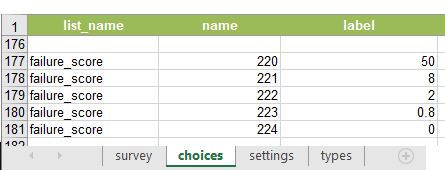# Calculation not working in Survey123

96
2
02-25-2020 02:10 AMNew Contributor

I have a multiplication calculation multiplying the answers to three select_one questions together. I (think!) I have set the bind::types right to make sure everything is treated as an integer. I get a number out of the calculation but it is orders of magnitudes off. For example it is calculating 2 x 20 x 10 as 13968240. Not sure how to fix it. I've tried doing the calculation under the calculate 'type' and as an interger 'type'. Relevant rows are highlighted.

Any help would be much appreciated!

Tags (2)
2 RepliesbyMVP Frequent Contributor

Try setting the bind::type field for the 3 fields going into the calculation not just the answer field.  I see you use the bind::esri one here instead.  Try to set all of them in bind::type to int.New Contributor III

castle head, using a calculator 222*242*260 equals number you gave, the calculations are done on the name column of your select_one list not the label. I end up giving the name and the title columns the same value so the forms display correctly and the math works out as expected